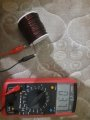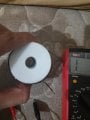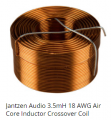# Measure inductance of air core inductor

#### Marcodavid

Joined Aug 1, 2022
39
I am making an inductor

- Air core
- Wire: 18 AWG guage
- Turns: (I am not sure ) but could be about 6000 turns
- The weight of the wire : 400 gram
- The coil wrapped by machine
- Coil lenght is 5 cm
- Diameter of core is 1.5 cm

I used an inductance meter to measure the inductance and I got a weird reading of this large inductor = 3.7mH.

The DC resistance also is wire = 3.5 ohm.

Could these measurements be correct although it is a large inductor?

If so, how can I increase the inductance to 150mH?#### Ian0

Joined Aug 7, 2020
5,885
Are you sure about 6000 turns? There seems to be about 50 on a layer, and probably not more than 10 layers.

#### ronsimpson

Joined Oct 7, 2019
2,258
I used an inductance meter to measure the inductance and I got a weird reading of this large inductor = 3.7mH.

If so, how can I increase the inductance to 150mH?
More turns! Approximately 6.3x more turns.

•Ian0

#### Dodgydave

Joined Jun 22, 2012
10,395
A rough guess at 700 turns should be 160mH..

#### crutschow

Joined Mar 14, 2008
30,455
Could these measurements be correct although it is a large inductor?
Yes. probably.
It may be large physically, but air core inductors have a much smaller inductance for a given number of turns compared to one with a magnetic core (iron, ferrite, etc.).
Here's a picture of a 3.5mH, 18 AWG air core inductor to show the relative size.So you need a much larger coil.

Why do yo want such a large air-core inductor?

#### ronsimpson

Joined Oct 7, 2019
2,258
Search for "inductor calculator". That will gave you an idea of Diameter, Turns, Length, Inductance.

#### MisterBill2

Joined Jan 23, 2018
12,704
Consider that the capacitive reactance of allthose turns in close proximity tends to cancel the inductive reactance. This is an unfortunate reality. So now comes the question about "what is the application for the inductance?"